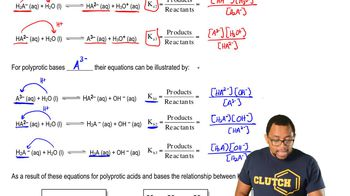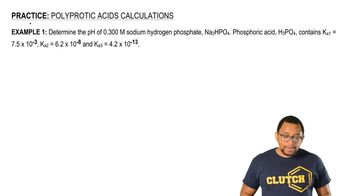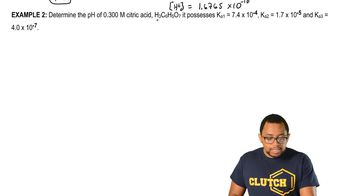Start typing, then use the up and down arrows to select an option from the list.# Polyprotic Acids

Jules Bruno
117views
So now let's take a look at the polic product base forms. So for the polic product based form is basically going the opposite direction. So the fully basic form when it has none of it's acidic hydrogen XYZ default a three minus. So here we're talking about accepting our first acidic hydrogen from water. Since we're talking about accepting that first H plus, we're dealing with a B one, so the equilibrium expression would be H A to minus times O H minus, divided by a three minus. Then that form could continue to accept H plus from another water molecule. So this would be dealing with accepting the second Age plus, so that bkb too. So we're gonna say here, that is H two A minus Oh h minus over h A to minus. And then finally, we accept the final H plus possible to turn into our fully acidic form at the end. So that be H three a times O. H minus, divided by H two a minus. So those would be the equilibrium. Expressions now realize here that when it comes to a try, product acid has basically four different versions of itself. So we have the acidic form or it has all three of its acidic hydrogen. Then we removed the first H plus from it. So we're gonna call this intermediate one. Then we could remove another H plus from it. We're gonna call this intermediate too, and then finally removed the last acidic hydrogen. And we're gonna say that this is the basic version or basic form, because it has none of it's acidic hydrogen. We're gonna say here removing the first acidic hydrogen means that we're dealing with K a one we're moving the second acidic hydrogen means we're dealing with a two. And then this would be K three in the opposite way. This basic form could start accepting H plus ions. So accepting that first a city hydrogen gives us K B one this accepting the second one means we're dealing with K B two. And finally this one here accepting the last acidic hydrogen possible gives us a K B three to give us this form. Okay, so you could either be looking at it going this way or going this way. And what you need to realize here is that K B one in K three are both connected together because they represent a connection between these two forms. Then you're gonna say here that K two and K B two are connected together because they connect these two forms. Then finally, K B three and K one are connected together because they give us these two forms here knowing that is important because that helps us go from one form to the other. So here, like I said, K one and K B three, or connect together and multiplying That gives us kw que two times K B two gives us kw and then K three and K B one gives us kw. Remember here that K W equals 1.0 times 10 to the negative 14 at 25 degrees Celsius. Even if they don't give you the temperature, you assume 25 degrees Celsius. Okay, w will be that number. If we change the temperature that changes are K w. Then they would have to tell you what the new K W value would be. But remember the connections between the different K A values and KB values when it comes to try product acids. Now that we've looked at the poly product acid forms and the poly protein basic forms. We can now look at how we approach calculating pH for these different versions of these compounds.03:0104:1204:0805:1806:56